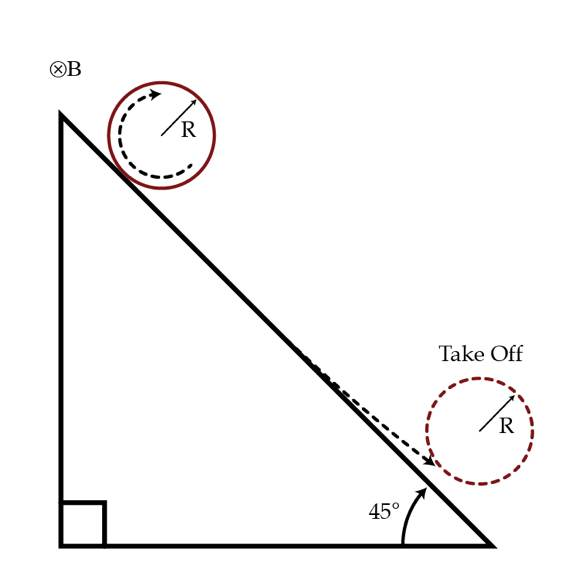# Magnetic takeoff

A thin charged loop with a charge $q>0$ (uniformly distributed) and mass $m$ is placed on a ramp which forms and angle $\alpha= 45^{\circ}$ with the horizontal. Due to the presence of an external homogeneous magnetic field of magnitude $B$ directed into the page, the loop "takes off" after a time $t^{*}$. Determine the time $t^{*}$ in seconds if $\frac{q B}{m}= 1~\mbox{s}^{-1}.$ Assume that the loop rolls without slipping while in contact with the ramp.×